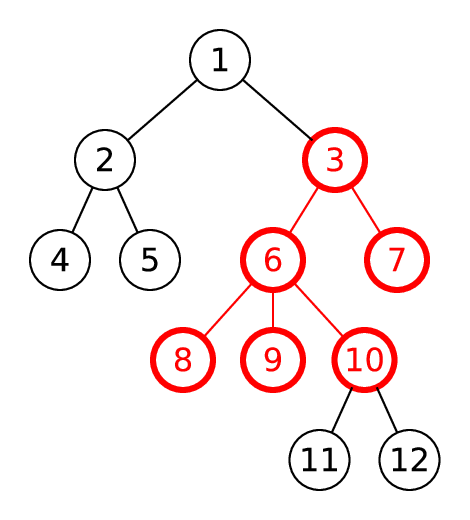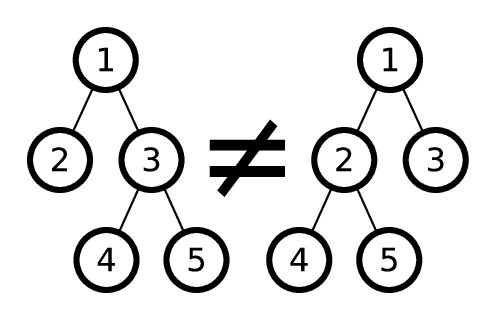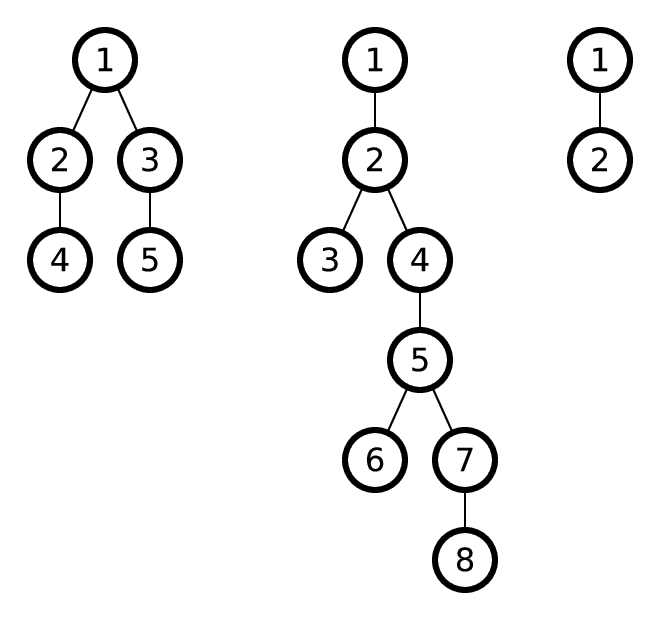시간 제한메모리 제한제출정답맞힌 사람정답 비율
4 초 512 MB10013917.647%

## 문제

Consider a rooted tree. Let us consider a vertex v that has at least one vertex in its subtree that has distance k from v. Let us call a tree that we get from a subtree rooted at v by removing all vertices that are at distance greater than k from v its k-subtree rooted at v. For example, the picture below shows 2-subtree of the vertex 3 in red.Let us call two subtrees equal, if it is possible to renumber vertices of one of them to get the other one. You are not allowed to change the order of children of a vertex. For example, the following two trees are not equal:You are given a rooted tree. Find the greatest k such that the given tree has two equal k-subtrees with different roots. These trees are allowed to have vertices in common.

The picture shows trees from sample test.The first example has equal 1-subtrees rooted at 2 and 3.

The second example has equal 3-subtrees rooted at 1 and 4.

The third example has equal 0-subtrees rooted at 1 and 2.

## 입력

Input data contains multiple test cases. The first line contains t — the number of tests (1 ≤ t ≤ 104).

Each of the following t test is described in the following way: the first line contains one integer n — the number of vertices (2 ≤ n ≤ 105).

The following n lines describe vertices, the i-th of them first contains an integer cnti — the number of vertices of the vertex i, followed by cnti integers — numbers of the children of the i-th vertex, in order. Vertices are numbered starting from 1. The root of the tree is the vertex number 1. It is guaranteed that the graph is the correct tree rooted at 1.

The sum of values of n for all tests in one input data doesn't exceed 2·105.

## 출력

For each test case output maximal k such that there are two equal k-subtrees with different roots.

## 예제 입력 1

3
5
2 2 3
1 4
1 5
0
0
8
1 2
2 3 4
0
1 5
2 6 7
0
1 8
0
2
1 2
0


## 예제 출력 1

1
3
0